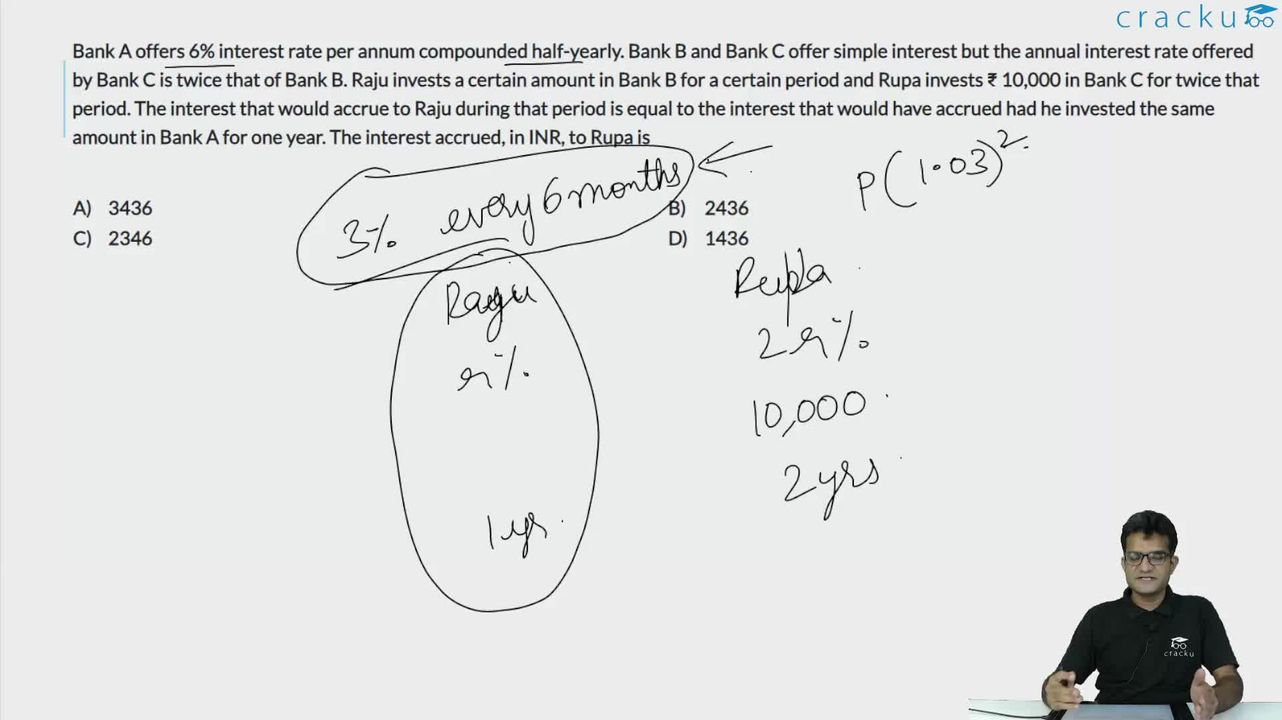Question 60

# Bank A offers 6% interest rate per annum compounded half-yearly. Bank B and Bank C offer simple interest but the annual interest rate offered by Bank C is twice that of Bank B. Raju invests a certain amount in Bank B for a certain period and Rupa invests ₹ 10,000 in Bank C for twice that period. The interest that would accrue to Raju during that period is equal to the interest that would have accrued had he invested the same amount in Bank A for one year. The interest accrued, in INR, to Rupa is

Solution

Bank A: 6% p.a. 1/2 yearly (CI)

Bank B: x% p.a (SI)

Bank C: 2x% p.a (SI)

Let Raju invest Rs P in bank B for t years. Hence, Rupa invests Rs 10,000 in bank C for 2t years.

Now,

$$P\left(\frac{x}{100}\right)t\ =\ P\left(1+\frac{3}{100}\right)^2-P$$

$$\left(\frac{x}{100}\right)t\ =\ 1.0609-1$$

$$\left(\frac{x}{100}\right)t\ =\ 0.0609$$

We need to calculate

SI = $$10000\times\ 2t\times\ \left(\frac{2x}{100}\right)=40000\left(\frac{x}{100}\right)t=40000\times\ 0.0609=2436$$

### View Video Solution